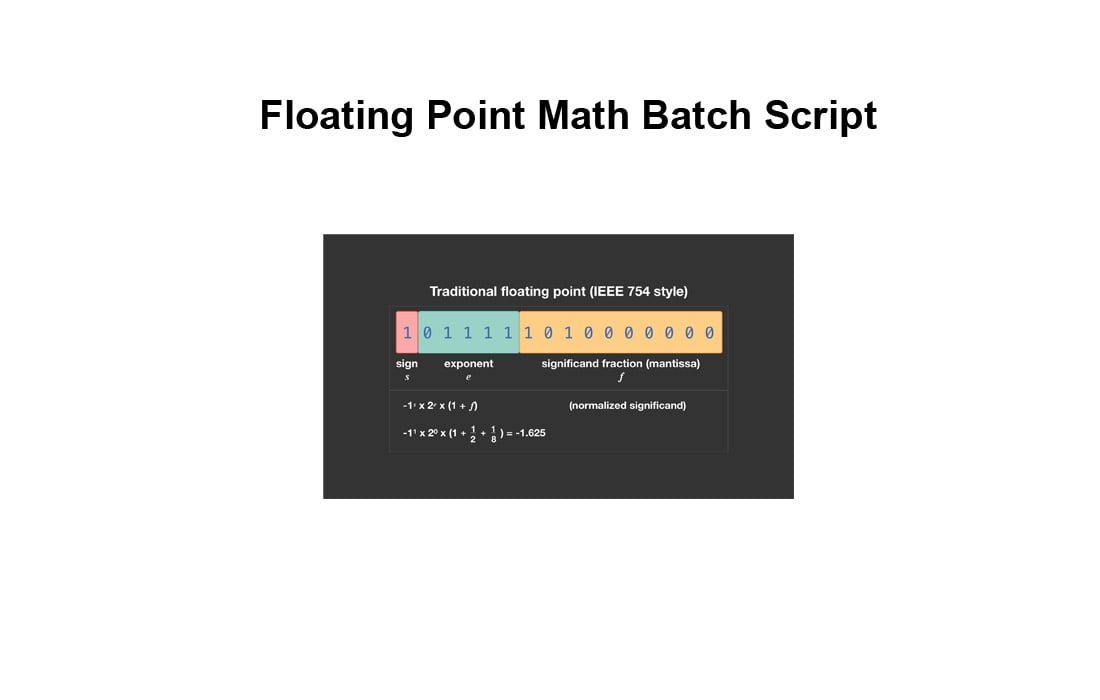# Floating Point Math Batch ScriptWell, no, it’s well documented that batch scripting cannot handle floating point maths.   However, by incorporating a few lines of VB scripting floating point math may be successful.

This hybrid batch/VB script shows how some calculations can easily be made.   The amounts used are for demonstration purposes only and it is not recommended that the script be used for sensitive currency calculations.   The script has been briefly tested in Win XP.

`@echo off`
`cls`
`setlocal`

`echo wscript.echo eval(wscript.arguments(0))>%temp%\eval1.vbs`

`set total=1182078.11`
`set figtwo=4.70`
`set figtre=17.5`

`:: Calculate 4.70% of 1182078.11 & store the result in environment variable Grint`
`set c=%total%*(%figtwo%/100)`
`call :calc`

`set grint=%result%`

`:: Deduct 17.5% of Grint`
`set c=%grint%-(%grint%*(%figtre%/100))`
`call :calc`

`set net=%result%`

`:: Add Net to the value in Total giving Newtot `
`set c=%total%+%net%`
`call :calc`

`set newtot=%result%`

`echo     Start bill = %total:~-10%`
`echo.`
`echo   Gross markup = %grint:~-10%`
`echo    Nett markup = %net:~-10%`
`echo.`
`echo       New bill = %newtot:~-10%  (Start bill + Nett markup)`

`del %temp%\eval1.vbs`
`exit /b`

`:calc`
`for /f %%A in ('cscript //nologo %temp%\eval1.vbs "round(%c%,2)"') do (`
`    set result=        %%A`
`)`